Binomial Distribution Sample Confidence Intervals Estimation

7. Absolute Risk Reduction and ARR-like Expressions

“Iuliu Haţieganu” University of Medicine and Pharmacy, Cluj-Napoca, Romania

sbolboaca@umfcluj.ro

Abstract

Assessments of a controlled clinical trial suppose to interpret some key parameters as the controlled event rate, experimental event date, relative risk,  absolute risk reduction, relative risk reduction, number needed to treat when the effect of the treatment are dichotomous variables. Defined as the difference in the event rate between treatment and control groups, the absolute risk reduction is the parameter that allowed computing the number needed to treat. The absolute risk reduction is compute when the experimental treatment reduces the risk for an undesirable outcome/event. In medical literature when the absolute risk reduction is report with its confidence intervals, the method used is the asymptotic one, even if it is well know that may be inadequate. The aim of this paper is to introduce and assess nine methods of computing confidence intervals for absolute risk reduction and absolute risk reduction – like function.

Computer implementations of the methods use the PHP language. Methods comparison uses the experimental errors, the standard deviations, and the deviation relative to the imposed significance level for specified sample sizes. Six methods of computing confidence intervals for absolute risk reduction and absolute risk reduction-like functions were assessed using random binomial variables and random sample sizes.

The experiments shows that the ADAC, and ADAC1 methods obtains the best overall performance of computing confidence intervals for absolute risk reduction.

Keywords

Confidence interval estimation; Absolute risk reduction; Absolute risk increase; Absolute benefit increase; Therapy studies assessment

Introduction

Assessments of the therapy studies suppose to interpret many key parameters including the absolute risk reduction, absolute risk increase, and absolute benefit increase when de results are dichotomous variables. The absolute risk reduction defined as ״the difference in the event rate between treatment group and control groups" ,  is the parameter which can be compute when the experimental treatment reduces the risk for an undesirable outcome/event. When the treatment harms more patients than the control treatment, the absolute risk increase is computes using the same formula as the one used for absolute risk reduction. When the experimental treatment increases, the chance of a desirable outcome/event the absolute benefit increase is obtain. If we looked at the mathematical formula of these parameters, all parameter described above have the same expression and, the expression represents the absolute differences between two proportions .

The confidence intervals for differences between two proportions were describe in a few papers , , , . There were not described differentiated the absolute and the relative differences between two proportion. Newcombe  recommend two methods based on Wilson method as the best method for computing confidence intervals for relative difference between two proportions. Unfortunately, the only method reported in medical literature for absolute risk reduction is the asymptotic method (called here ADWald) which is well knowing that may be inadequate .

The aim of this paper is to introduce and assess nine methods of computing confidence intervals for absolute risk reduction and absolute risk reduction-like functions.

Materials and Methods

When the results of a therapy are dichotomous variables a 2´2 contingency table can be create, table which contain four groups of cases, noted usually with a, b, c, and d. The real positive cases (patients which receive the new treatment and the treatment was efficient), usually noted with a, false positive cases (patients which receive the new treatment and the treatment was not efficient), usually noted here with b. The false negative cases (patients which receive an old treatment and the treatment was efficient), usually noted with c and true negative cases (patients which receive an old treatment and the treatment was not efficient), noted usually with d.

Using the following substitutions: a = Y, b = n-Y, c = X, d = m-X (where X and Y are independent binomial distribution variables of sizes m and n) the absolute risk reduction (ARR) becomes: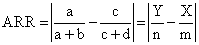(1)

From the mathematical point of view, the absolute risk increase (ARI) and the absolute benefit increase (ABI) have the same formulas but they can be calculate in different situation as was present in Introduction. Thus, mathematical speaking, the absolute risk reduction, and ARR-like functions dependency is of |Y/n-X/m| function-type. Let us call ci6 the |Y/n-X/m| expression, as were define in a previous paper .(2)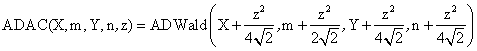(3)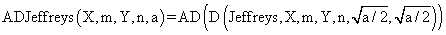(4)(5)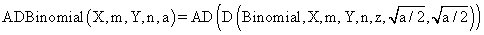(6)(7)(8)(9)(10)(11)

The above-described functions were implements into a PHP program. The source codes for the functions are:

\$t0 = \$z * pow(\$X*(\$m-\$X)/pow(\$m,3)+\$Y*(\$n-\$Y)/pow(\$n,3),0.5);

return array( \$tXi , \$tXs ); }

\$Y+pow(\$z,2)/4/pow(2,0.5),\$n+pow(\$z,2)/2/pow(2,0.5),\$z,\$a);}

/pow(\$m,2))),\$Y+0.5*(1-2*sqrt(\$Y*(\$n-\$Y)/pow(\$n,2))),\$n+

+1*(1-2*sqrt(\$Y*(\$n-\$Y) /pow(\$n,2))),\$z,\$a);}

\$t0 = \$z * pow(\$X*(\$m-\$X)/pow(\$m,3)+\$Y*(\$n-\$Y)/pow(\$n,3)+2*(1+2/pow(\$m*\$n,0.5))

return array( \$tXi , \$tXs ); }

\$t0 = \$z * pow(\$X*(\$m-\$X)/pow(\$m,3)+\$Y*(\$n-\$Y)/pow(\$n,3)

return array( \$tXi , \$tXs ); }

\$t0 = \$z * pow(\$X*(\$m-\$X)/pow(\$m,3)+\$Y*(\$n-\$Y)/pow(\$n,3)+

return array( \$tXi , \$tXs ); }

(1-0.125/log(\$m))));    }

In order to obtain a 100·(1-α) = 95% confidence intervals (is most frequently used confidence intervals) the experiments were runs at a significance level α = 5% (noted with a in our program). Corresponding to choused significance level was used its normal distribution percentile z1-α/2 = 1.96 (noted with z in our program). The sequence of the program is :

define("z",1.96); define("a",0.05);

In the assessment of the confidence intervals methods were used two formulas for computing the standard deviations, one which correspond to the standard deviation and the other one which correspond to the deviation relative to the imposed significance level α = 5% (see paper ).

The performance of each method for different sample sizes (m, n) and different values of binomial variables (X, Y) were asses using a set of criterions. First were evaluates the upper and lower limits for a given X, Y and an equal sample size (m = n= 50) for specified methods (ADJeffreys and ADAC):

define("N_min",50);define("N_max",51);est_ci2_er(z,a,\$c_i,"ci6","ci");

Second, the assessment of the percentages of the experimental errors and standard deviations, for representative values for a list of equal (m = n) sample sizes were performs:

·        For n = 10: define("N_min",10); define("N_max",11); est_ci2_er(z,a,\$c_i,"ci6","er");

·        For n = 20 was modified as follows: define("N_min",20);define("N_max",21);

·        For n = 30 was modified as follows: define("N_min",30); define("N_max",31);

The standard deviation of the experimental error (StdDev) was computes using the next formula:(12)

where StdDev(X) is standard deviation, Xi is the experimental errors for a given i, M(X) is the arithmetic mean of the experimental errors and n is the sample size.

If we have a sample of n elements with a known (or expected) mean (equal with 100α), the deviation around α = 5% (imposed significance level) is giving by:(13)

Third, the assessment of the experimental errors and standard deviations at central point X = Y and m = n = 4, 6..204 (m, n even numbers) was carry on. The sequence of the program, which allowed us to compute the percentages of the experimental errors, is:

define("N_min", 2); define("N_max",205); est_C2(z,a,\$c_i,"ci6");

The dependences of the experimental errors average and of the deviations relative to the imposed significance level (α = 5%) for m = 4..14 and n = 4..14 were compute using the next sequence of the program:

define("N_min", 4); define("N_max",15); est_ci2_er (z,a,\$c_i,"ci6", "mv");

The last part of the experiment consisted on assessing the performance of methods in 100 random binomial variables X, Y (1 ≤ X, Y < m, n) and random sample sizes m, n (4 ≤ m, n ≤ 1000):

define("N_min", 4); define("N_max",1000); est_ci2_er(z,a,\$c_i,"ci6","ra");

We did not have the resources needed to perform the experiment for the methods that were based on the hypothesis of binomial distribution (ADJeffreys, ADJeffreyC, ADBinomial, ADBinomialC).

Results

The confidence intervals limits for ci6 function at m = n = 50 were obtained and the results were graphical represents using the SlideWrite Plus program (figure 1) and Microsoft Excel (figure 2).

The Slide graphical representations (figure 1) were create using a 3D-Mesh graph type with 80% perspective, 45° tilt angle and 45° rotation angle. On X-axis was represents the values of X, on the Y-axis the values of Y and on the Z-axis the values of ci6 function, or the lower and the upper confidence intervals limits. There were represent with red color the experimental values from 0 to 2, with green the values from 2 to 4, with blue the values from 4 to 6, with cyan the values from 6 to 8, and with magenta the values from 8 to 10.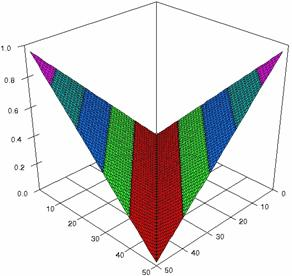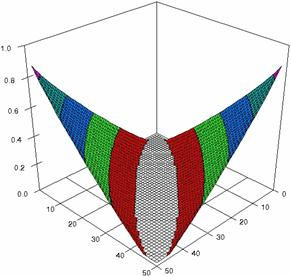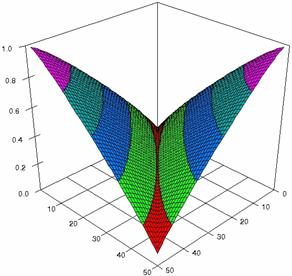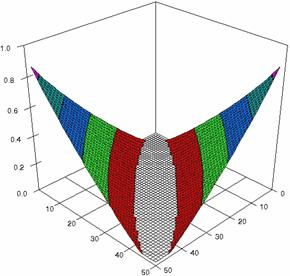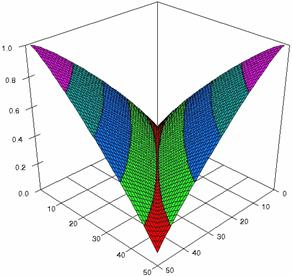Figure 1. The representations of the ci6 function and its confidence intervals limits computed with ADJeffreysC and ADAC at 0 < X, Y < m = n = 50

In figure 2 the confidence limits were represents depending on the values of the ci6 function for m = n = 50 using ADJeffreysC and ADAC methods.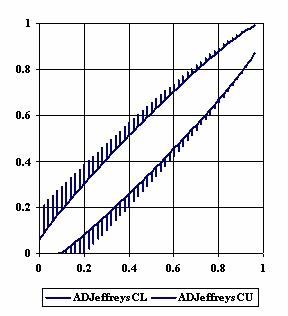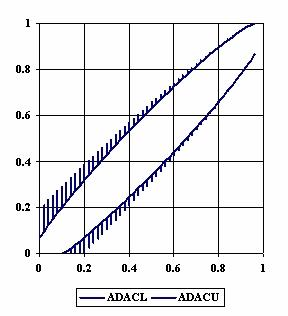Figure 2. The upper and lower confidence limits for ci6 function

with ADJeffreysC, and ADAC at 0 < X, Y < m = n = 50

The contour plots of percentages of the experimental errors for equal sample sizes (m = n = 10, 20, 40) were illustrate in figure 3-5. On X-axis were represent the values of X binomial variable, on Y-axis the values of Y binomial variable, and on Z-axis the percentages of experimental errors for each specified method. The graphical representations were create using a 3D-Mesh graph type with 80% perspective, 45° tilt angle and 45° rotation angle. On the plots were represent the percentages of the experimental errors with red color (0-2%), green (2-4%), blue (4-6%), cyan (6-8%), and magenta (8-10%).

The graphical representations of the experimental errors percentages for m = n = 10 were presented in figure 3; for m = n = 20 in figure 4, and for m = n = 30 in figure 5.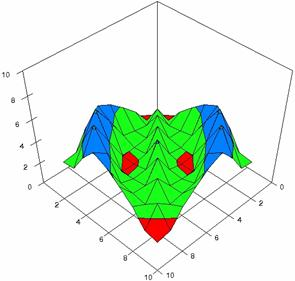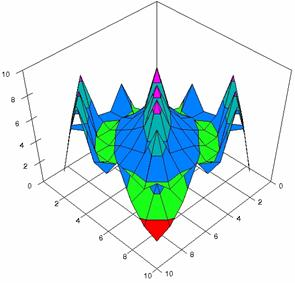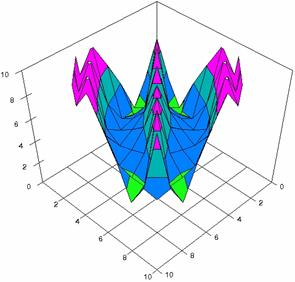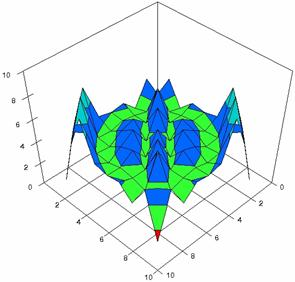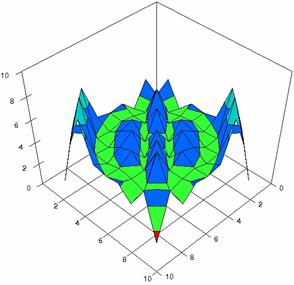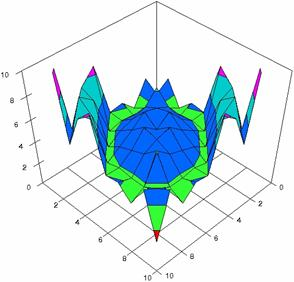Figure 3. The percentages of experimental errors for ci6 function with ADAC, ADAC1,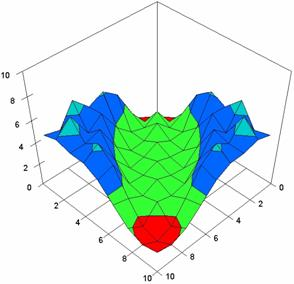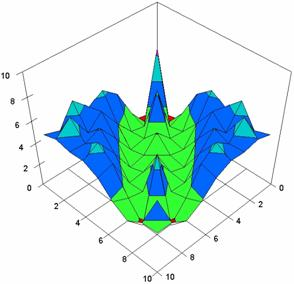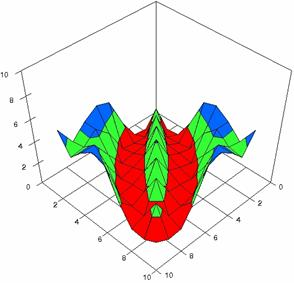Figure 3. The percentages of experimental errors for ci6 function  with ADJeffreys, ADJeffreysC, ADBinomial, and ADBinomialC at 0 < X, Y < m = n = 10

The averages of experimental errors (MErr) and standard deviations (StdDev) for ci6 function using all the method are in table 1.

 m=n ADAC ADAC1 ADWald ADAs0 ADAs1 10 3.10 (1.36) 4.63 (1.95) 7.00 (3.60) 4.24 (1.40) 4.24 (1.40) 20 3.75 (1.38) 4.35 (1.77) 5.74 (2.60) 4.71 (1.45) 4.36 (1.44) 30 5.28 (2.04) 4.42 (1.45) 5.28 (2.04) 3.84 (1.37) 4.62 (1.40) m=n ADAs2 ADJeffreys ADJeffreysC ADBinomial ADBinomialC 10 5.13 (1.99) 3.78 (1.95) 4.29 (2.20) 2.43 (1.58) 2.53 (1.59) 20 4.95 (1.68) 4.44 (1.72) 4.72 (1.72) 3.60 (1.61) 3.87 (1.65) 30 4.41 (1.37) 4.74 (1.48) 4.47 (1.73) 4.84 (1.84) 3.98 (1.63)

Table 1. The MErr and StdDev (into parentheses) for ci6 function at m = n = 10, 20, and 30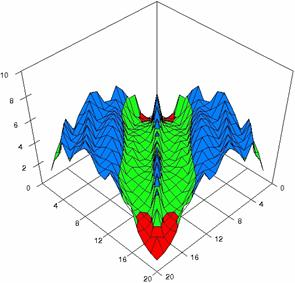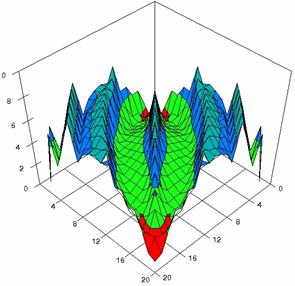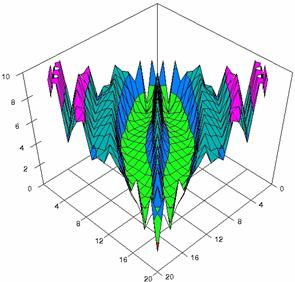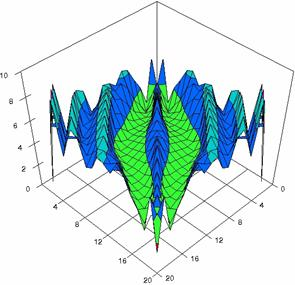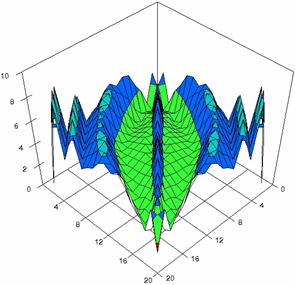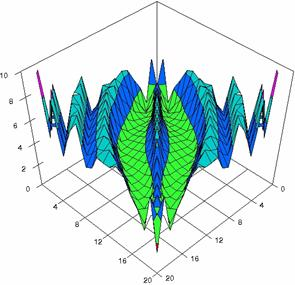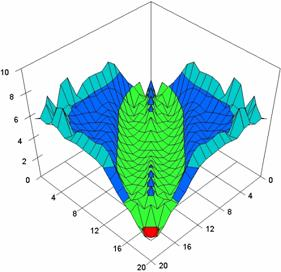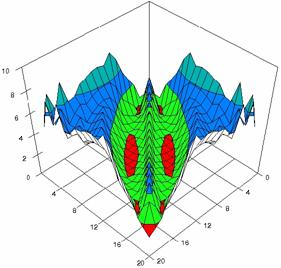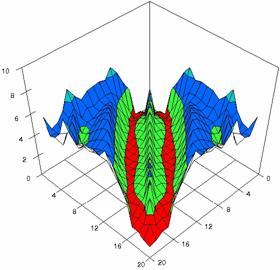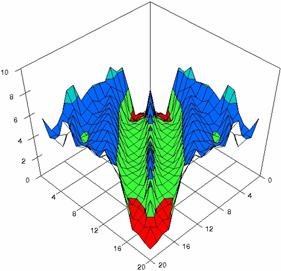Figure 4. The percentages of experimental errors for ci6 function with ADJeffreys, ADJeffreysC, ADBinomial and ADBinomialC at 0 < X, Y < m = n = 20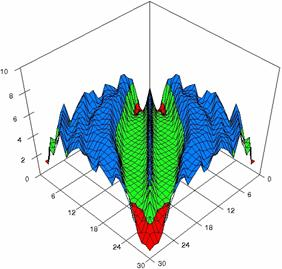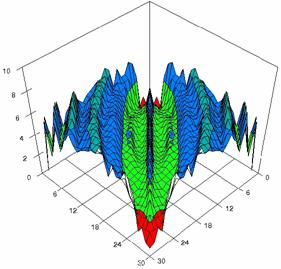Figure 5. The percentages of experimental errors for ci6 with ADAC, and ADAC1 at m=n=30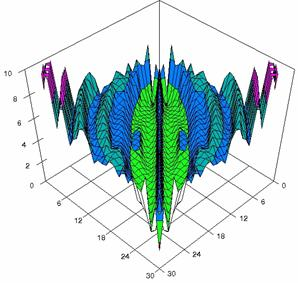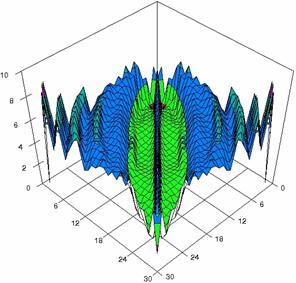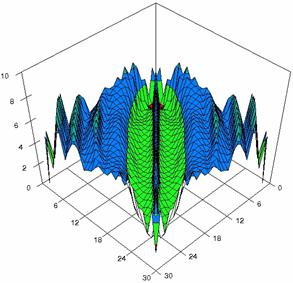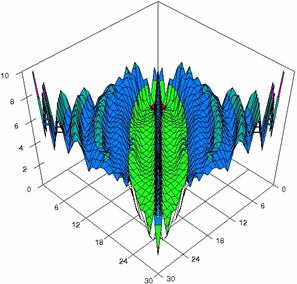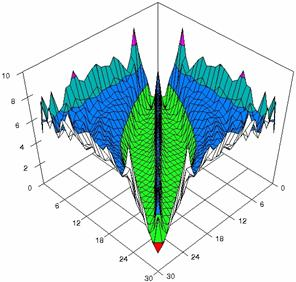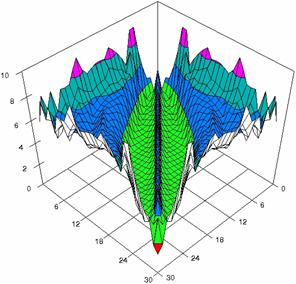Figure 5. The percentages of experimental errors for ci6 function with ADWald, ADAs0, ADAs1, ADAs2, ADJeffreys, and ADJeffreysC at 0 < X, Y < m = n = 30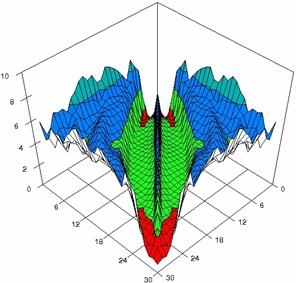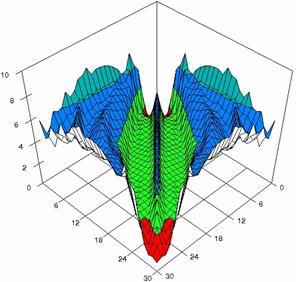Figure 5. The percentages of experimental errors for ci6 function with ADBinomial, and ADBinomialC at 0 < X, Y < m = n = 30

The assessment of the confidence intervals methods was carry on with a particular situation: X = Y and m = n = 4, 6,..204 (even number).

The experimental results were import in Microsoft Excel where the graphical representations were creating (figure 6). On horizontal-axis were represent the m = n = 4, 6..204 (even number) depending on X = Y and on the vertical axis the percentages of the experimental errors.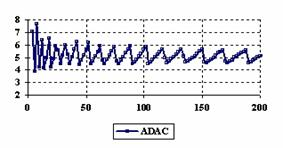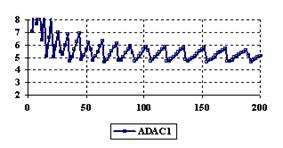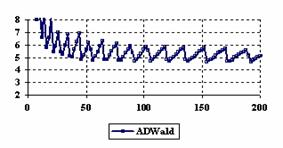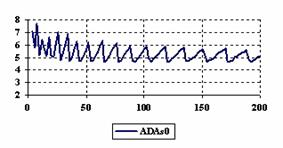Figure 6. The percentages of the experimental errors for ci6 function

at X = Y and m = n = 4,6..200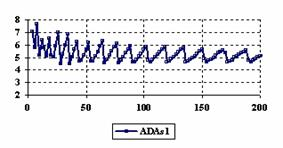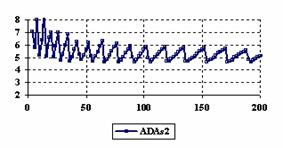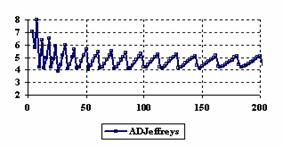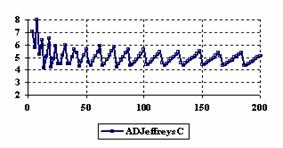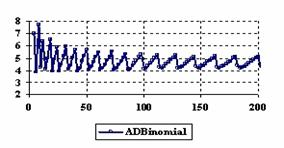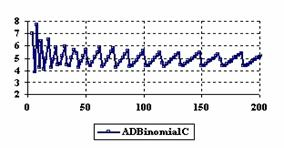Figure 6. The percentages of the experimental errors for ci6 function

at X = Y and m = n = 4,6..200

The averages (MErr) and standard deviations (StdDev) of the experimental errors were present in table 2.

Table 2. The averages and standard deviations of the experimental errors

for ci6 function at central point (X=Y) and m = n (4,6..204)

The error maps of dependencies of averages of the experimental errors (left side graphs) and of the deviations relative to significance level α = 5% (right side graphs) for m = 4..14 and n = 4..14 were present in figure 7.

The error maps were creates with 80% perspective, 30° tilt angle, and 45° rotation angle. For the graphical representation of the experimental errors (left side graphics), with red color were represent the experimental percentages from 0 to 2%, with green from 2 to 4%, with blue from 4 to 6%, with cyan from 6 to 8%, and with magenta from 8 to 10%.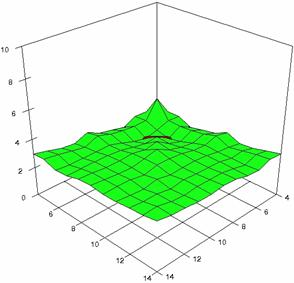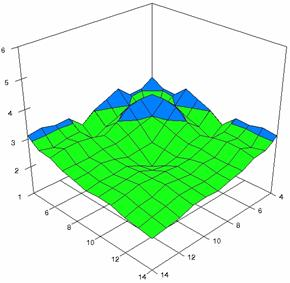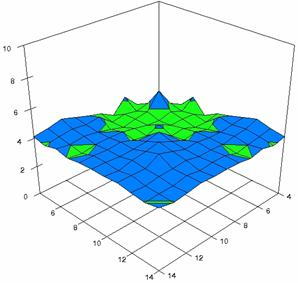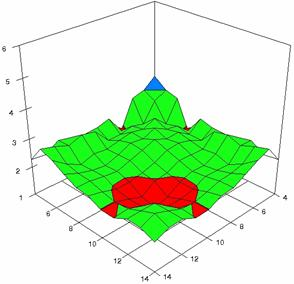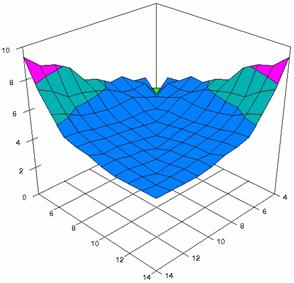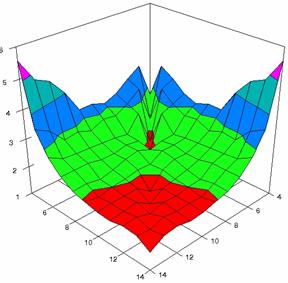Figure 7.  Averages of experimental errors (left) and deviations relative to the significance level α = 5% (right) with ADAC, ADAC1, and ADAs0 at m, n = 4..14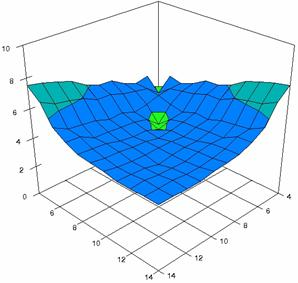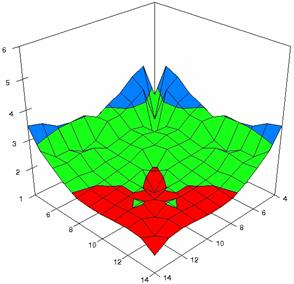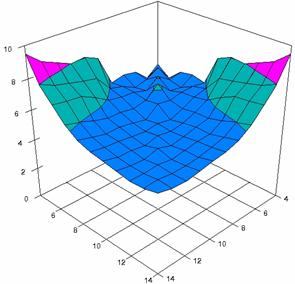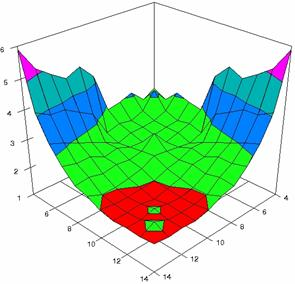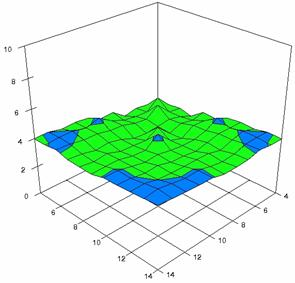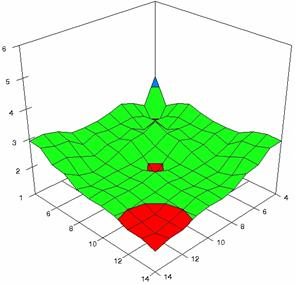Figure 7. Averages of experimental errors (left) and deviations relative to the significance level α = 5% (right) with ADAs1, ADAs2, ADJeffreys at m, n = 4..14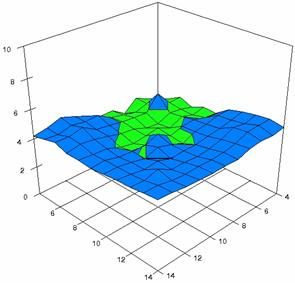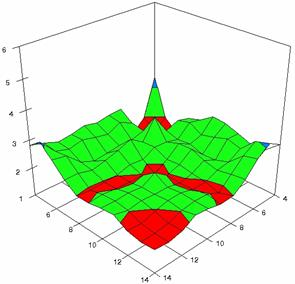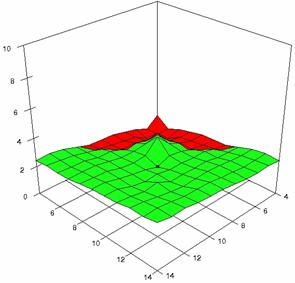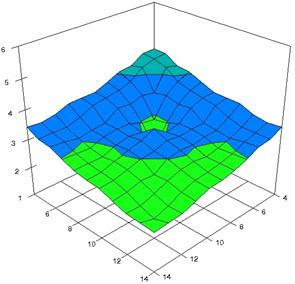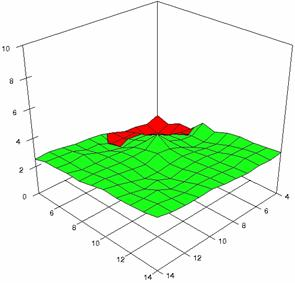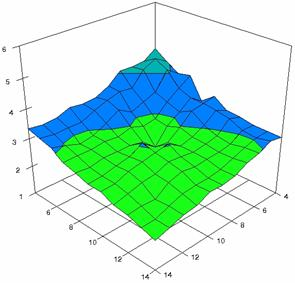Figure 7. Averages of experimental errors (left) and deviations relative to the significance level α = 5% (right) with ADJeffreysC, ADBinomial, and ADBinomialC at m, n = 4..14

In the right side graphs (figure 7) were represented with red color the values from 1 to 2, with green color the values from 2 to 3, with blue the values from 3 to 4%, with cyan the values from 4 to 5, with magenta the values from 5 to 6, and with yellow the values from 6 to 7. The averages of the experimental errors (MErr) and the deviations relative to the imposed significance level α = 5% (Dev5) for sample sizes (m, n) which vary in 4..14 domain are present in table 3.

Table 3. The averages of experimental errors and deviations relative to imposed significance level for ci6 function when sample sizes m, n vary in 4..14 domain

Using the results obtained from the 100 random binomial variable (X, Y; 1 ≤ X < m, and 1 ≤ Y < n) and samples size (m, n) from 4 to 1000 domain, a set of calculations as are described in paper  are done and presented in tables 4-7 and represented in figure 8.

Table 4 contains the deviations of the experimental errors relative to the imposed significance level (Dev5), the absolute differences of the average of experimental errors relative to the imposed significance level (|5-M|), and standard deviations (StdDev) of the experimental errors.

Table 4. Methods ordered by performance according to Dev5, |5-M| and StdDev criterions

In the figure 8 were represents with black dots the frequencies of the experimental error for each specified method; with green line the best errors interpolation curve with a Gauss curve (dIG(er)). The Gauss curves of the average and standard deviation of the experimental errors (dMV(er)) were represented with red line, while the Gauss curve of the experimental errors deviations relative to the significance level (d5V(er)) with blue squares. The Gauss curves of the standard binomial distribution from the average of the errors equal with 100·α (pN(er,10)) were represent with black line.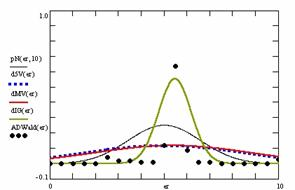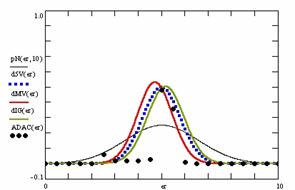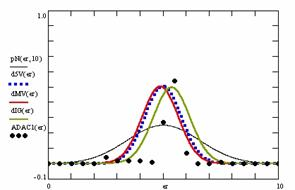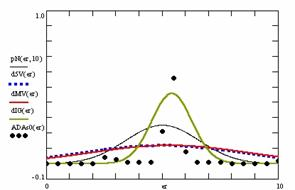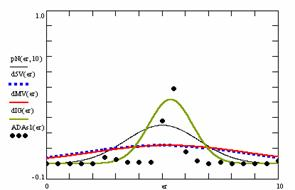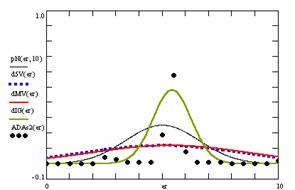Figure 8. The pN(er, 10), d5V(er), dMV(er), dIG(er) and the frequencies of the experimental errors for each specified method for random X, m, Y, n

Table 5. The methods ordered by |5-Mint|, DevInt, r2Int and FInt criterions

Table 5 contains the absolute differences of the averages that result from Gaussian interpolation curve to the imposed significance level (|5-MInt|), the deviations that result from Gaussian interpolation curve (DevInt), the correlation coefficient of interpolation (r2Int) and the Fisher point estimator (FInt).

The superposition between the standard binomial distribution curve and interpolation curve (pNIG), the standard binomial distribution curve and the experimental error distribution curve (pNMV), and the standard binomial distribution curve and the error distribution curve around significance level (pN5V) are present in table 6.

Table 6. Methods ordered by the pNING, pNMV, and pN5V criterions

Table 7 contains the percentages of superposition between interpolation Gauss curve and the Gauss curve of error around experimental mean (pIGMV), between the interpolation Gauss curve and the Gauss curve of error around imposed mean (α = 5%) (pIG5V), and between the Gauss curve experimental error around experimental mean and the error Gauss curve around imposed mean α = 5% (pMV5V).

Table 7. The confidence intervals ordered by the pIGMV, pIG5V, and pMV5V criterions

Discussions

The graphical representation of the experimental confidence limits for specified equal sample sizes (m = n) and specified method of computing confidence intervals is not a useful criterion of assessment. As can be seen from figure 1 and 2 we could not say that there were significant differences between ADJeffreysC and ADAC methods.

Looking at the results from the central point experiment (X = Y, and m = n = 4, 6..2004) we can observed that the ADJeffreysC method closely followed by the ADAC and ADBinomialC methods obtained the experimental errors averages closest to the imposed significance level (α = 5%). For the central point estimation there were two methods represented by the ADWald and ADAC1 that present for the extreme values of the binomial variables (X = Y) averages of the experimental errors greater than 8%. This behavior can be seen until X = Y = 5.

Analyzing the results obtained for sample sizes vary in 4..14 domain (m = 4..14, n = 4..14) we can observe that, excepting the ADAs2 method all the averages of experimental errors were less than expected value (100·α). The ADAs1 method was the one that obtained the best estimation (an average of the errors equal with 4.89%), followed by the ADJeffreysC method (4.18%). The lowest standard deviation relative to the imposed significance level (α = 5%) was obtained by the ADJeffreysC method (2.23).

Looking at the results obtained from the random samples (m, n) and binomial variables (X, Y) we can remark that the ADAC and ADAC1 methods obtained the closest experimental errors average to the imposed significance levels (α = 5%), the lowest experimental standard deviation, and the lowest deviation relative to the imposed significance level (α = 5%).

The ADAs1 method obtained the maximum superposition between the curve of interpolation and the curve of standard binomial distribution. The maximum superposition between the curve of standard binomial distribution and the curve of experimental errors was obtains by the ADAC1 method. The ADAC and the ADAC1 methods obtained the maximum superposition between the curve of standard binomial distribution and the curve of errors around the significance level (α = 5%).

The maximum superposition between the Gauss curve of interpolation and the Gauss curve of errors around experimental mean and the maximum superposition between the Gauss curve of interpolation and the Gauss curve of errors around significance level (α = 5%) was obtains by the ADAC method. The ADAC1 method obtained the maximum superposition between the Gauss curve of experimental errors and the Gauss curve of error distribution around imposed mean (α = 5%), followed by the ADAC method.

Conclusions

The percentages of the experimental errors for some methods of computing confidence intervals (ADAC, ADAs1, ADJeffreys, ADBinomial, ADBinomial) at equal sample sizes (m = n) increase with increasing of the samples sizes while for other methods (ADAC, ADWald, ADAs2) decrease with increasing of the sample size.

When the sample sizes vary from 4 to 14, the ADJeffreysC method obtained the best performances in computing confidence intervals for absolute risk reduction.

In case of random binomial variables (X, Y) and random sample sizes (m, n) all presented methods obtained for a specific task performance, but the ADAC and ADAC1 methods systematically obtained performance in estimating the confidence intervals for absolute risk reduction and absolute risk reduction like-functions.

Based on the above conclusions we recommend the ADAC, ADCA1 and ADJeffreysC functions as methods of computing confidence intervals for absolute risk reduction and absolute risk reduction-like functions.

Acknowledgements

First author is thankful for useful suggestions and all software implementations to Ph.D. Sci., M.Sc. Eng. Lorentz JÄNTSCHI from Technical University of Cluj-Napoca.

References

. Sackett D., Straus E.S., Richardson W.S., Rosenberg W., Haynes R.B., Therapy chapter from Evidence-based Medicine: How to Practice and Teach EBM, Second Ed., Edinburgh, Churchill Livingstone, 2000, p. 105-154.

. Klardie K.A., Johnson J., McNaughton M.A., Meyers W., Integrating the principles of evidence-based practice into clinical practice, J. Am. Acad. Nurse Practitioners, 2004, 16, p. 98, p. 100-2, p. 104-5.

. Drugan T., Bolboacă S., Jäntschi L., Achimaş Cadariu A., Binomial Distribution Sample Confidence Intervals Estimation 1. Sampling and Medical Key Parameters Calculation, Leonardo Electronic Journal of Practices and Technologies, 2003, 3, p. 45-74.

. Newcombe R.G., Two-sided confidence intervals for the single proportion; comparison of several methods, Statistics in Medicine, 1998, 17, p. 857–872.

. Agresti A., Dealing with discreteness: making ‘exact’ confidence intervals for proportions, differences of proportions, and odds ratios more exact. Statistical Methods in Medical Research, 2003, 12, p. 3-21.

. Rosner B., Hypothesis Testing: Categorical Data Chapter in Fundamentals of Biostatistics, Forth Edition, Duxbury Press, Belmont, 1995, p. 345-442.

Oxford Medical Publications, 1996, p. 127-130.

. Daly L.E., Confidence limits made easy: Interval estimation using a substitution method, American Journal of Epidemiology, 1998, 147, p. 783-790.

. Bolboacă S., Achimaş Cadariu A., Binomial Distribution Sample Confidence Intervals Estimation 2. Proportion-like Medical Parameters, Leonardo Electronic Journal of Practices and Technologies, 2003, 3, p. 75-110.

. Bolboacă S., Achimaş Cadariu A., Binomial Distribution Sample Confidence Intervals Estimation 5. Odds Ration, Leonardo Journal of Sciences, 2004, 4, p. 26-42.